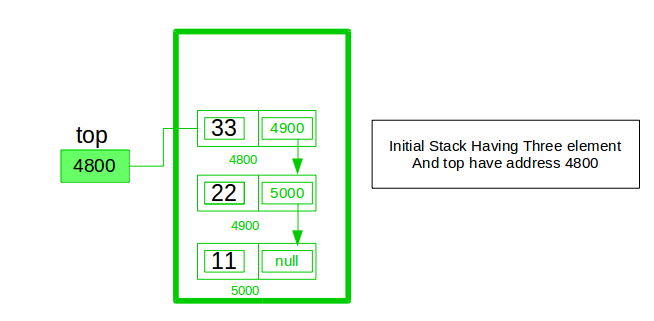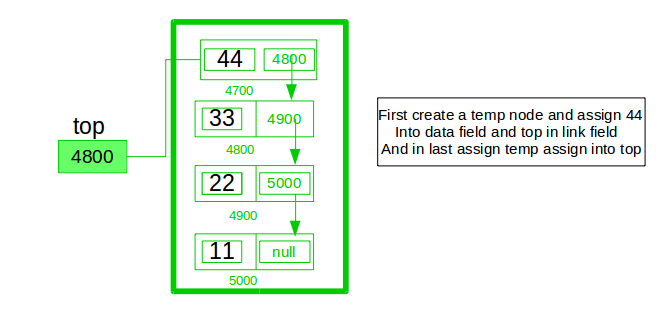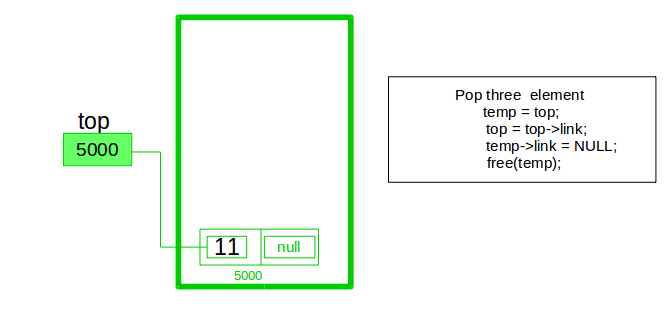# Implement a stack using singly linked list

• Difficulty Level : Easy
• Last Updated : 24 Jun, 2022

To implement a stack using singly linked list concept , all the singly linked list operations are performed based on Stack operations LIFO(last in first out) and with the help of that knowledge we are going to implement a stack using single linked list. Using singly linked lists , we implement stack by storing the information in the form of nodes and we need to follow the stack rules and implement using singly linked list nodes . So we need to follow a simple rule in the implementation of a stack which is last in first out and all the operations can be performed with the help of a top variable .Let us learn how to perform Pop , Push , Peek ,Display operations in the following article .A stack can be easily implemented using the linked list. In stack Implementation, a stack contains a top pointer. which is “head” of the stack where pushing and popping items happens at the head of the list. First node have null in link field and second node link have first node address in link field and so on and last node address in “top” pointer.
The main advantage of using linked list over an arrays is that it is possible to implement a stack that can shrink or grow as much as needed. In using array will put a restriction to the maximum capacity of the array which can lead to stack overflow. Here each new node will be dynamically allocate. so overflow is not possible.
Stack Operations:

1. push() : Insert a new element into stack i.e just inserting a new element at the beginning of the linked list.
2. pop() : Return top element of the Stack i.e simply deleting the first element from the linked list.
3. peek(): Return the top element.
4. display(): Print all elements in Stack.Below is the implementation of the above approach:

## C++

 `// C++ program to Implement a stack``//using singly linked list``#include ``using` `namespace` `std;`` ` `// Declare linked list node`` ` `struct` `Node``{``    ``int` `data;``    ``Node* link;``};`` ` `Node* top;`` ` `// Utility function to add an element``// data in the stack insert at the beginning``void` `push(``int` `data)``{``     ` `    ``// Create new node temp and allocate memory in heap``    ``Node* temp = ``new` `Node();`` ` `    ``// Check if stack (heap) is full.``    ``// Then inserting an element would``    ``// lead to stack overflow``    ``if` `(!temp)``    ``{``        ``cout << ``"\nStack Overflow"``;``        ``exit``(1);``    ``}`` ` `    ``// Initialize data into temp data field``    ``temp->data = data;`` ` `    ``// Put top pointer reference into temp link``    ``temp->link = top;`` ` `    ``// Make temp as top of Stack``    ``top = temp;``}`` ` `// Utility function to check if``// the stack is empty or not``int` `isEmpty()``{``    ``//If top is NULL it means that ``    ``//there are no elements are in stack``    ``return` `top == NULL;``}`` ` `// Utility function to return top element in a stack``int` `peek()``{``     ` `    ``// If stack is not empty , return the top element``    ``if` `(!isEmpty())``        ``return` `top->data;``    ``else``        ``exit``(1);``}`` ` `// Utility function to pop top``// element from the stack``void` `pop()``{``    ``Node* temp;`` ` `    ``// Check for stack underflow``    ``if` `(top == NULL)``    ``{``        ``cout << ``"\nStack Underflow"` `<< endl;``        ``exit``(1);``    ``}``    ``else``    ``{``         ` `        ``// Assign top to temp``        ``temp = top;`` ` `        ``// Assign second node to top``        ``top = top->link;`` ` `        ``//This will automatically destroy ``        ``//the link between first node and second node`` ` `        ``// Release memory of top node``        ``//i.e delete the node``        ``free``(temp);``    ``}``}`` ` `// Function to print all the``// elements of the stack``void` `display()``{``    ``Node* temp;`` ` `    ``// Check for stack underflow``    ``if` `(top == NULL)``    ``{``        ``cout << ``"\nStack Underflow"``;``        ``exit``(1);``    ``}``    ``else``    ``{``        ``temp = top;``        ``while` `(temp != NULL)``        ``{`` ` `            ``// Print node data``            ``cout << temp->data << ``"-> "``;`` ` `            ``// Assign temp link to temp``            ``temp = temp->link;``        ``}``    ``}``}`` ` `// Driver Code``int` `main()``{``     ` `    ``// Push the elements of stack``    ``push(11);``    ``push(22);``    ``push(33);``    ``push(44);`` ` `    ``// Display stack elements``    ``display();`` ` `    ``// Print top element of stack``    ``cout << ``"\nTop element is "``        ``<< peek() << endl;`` ` `    ``// Delete top elements of stack``    ``pop();``    ``pop();`` ` `    ``// Display stack elements``    ``display();`` ` `    ``// Print top element of stack``    ``cout << ``"\nTop element is "``        ``<< peek() << endl;``         ` `    ``return` `0;``}`

## Java

 `// Java program to Implement a stack``// using singly linked list``// import package``import` `static` `java.lang.System.exit;`` ` `// Create Stack Using Linked list``class` `StackUsingLinkedlist {`` ` `    ``// A linked list node``    ``private` `class` `Node {`` ` `        ``int` `data; ``// integer data``        ``Node link; ``// reference variable Node type``    ``}``    ``// create global top reference variable global``    ``Node top;``    ``// Constructor``    ``StackUsingLinkedlist()``    ``{``        ``this``.top = ``null``;``    ``}`` ` `    ``// Utility function to add an element x in the stack``    ``public` `void` `push(``int` `x) ``// insert at the beginning``    ``{``        ``// create new node temp and allocate memory``        ``Node temp = ``new` `Node();`` ` `        ``// check if stack (heap) is full. Then inserting an``        ``//  element would lead to stack overflow``        ``if` `(temp == ``null``) {``            ``System.out.print(``"\nHeap Overflow"``);``            ``return``;``        ``}`` ` `        ``// initialize data into temp data field``        ``temp.data = x;`` ` `        ``// put top reference into temp link``        ``temp.link = top;`` ` `        ``// update top reference``        ``top = temp;``    ``}`` ` `    ``// Utility function to check if the stack is empty or not``    ``public` `boolean` `isEmpty()``    ``{``        ``return` `top == ``null``;``    ``}`` ` `    ``// Utility function to return top element in a stack``    ``public` `int` `peek()``    ``{``        ``// check for empty stack``        ``if` `(!isEmpty()) {``            ``return` `top.data;``        ``}``        ``else` `{``            ``System.out.println(``"Stack is empty"``);``            ``return` `-``1``;``        ``}``    ``}`` ` `    ``// Utility function to pop top element from the stack``    ``public` `void` `pop() ``// remove at the beginning``    ``{``        ``// check for stack underflow``        ``if` `(top == ``null``) {``            ``System.out.print(``"\nStack Underflow"``);``            ``return``;``        ``}`` ` `        ``// update the top pointer to point to the next node``        ``top = (top).link;``    ``}`` ` `    ``public` `void` `display()``    ``{``        ``// check for stack underflow``        ``if` `(top == ``null``) {``            ``System.out.printf(``"\nStack Underflow"``);``            ``exit(``1``);``        ``}``        ``else` `{``            ``Node temp = top;``            ``while` `(temp != ``null``) {`` ` `                ``// print node data``                ``System.out.printf(``"%d->"``, temp.data);`` ` `                ``// assign temp link to temp``                ``temp = temp.link;``            ``}``        ``}``    ``}``}``// main class``public` `class` `GFG {``    ``public` `static` `void` `main(String[] args)``    ``{``        ``// create Object of Implementing class``        ``StackUsingLinkedlist obj = ``new` `StackUsingLinkedlist();``        ``// insert Stack value``        ``obj.push(``11``);``        ``obj.push(``22``);``        ``obj.push(``33``);``        ``obj.push(``44``);`` ` `        ``// print Stack elements``        ``obj.display();`` ` `        ``// print Top element of Stack``        ``System.out.printf(``"\nTop element is %d\n"``, obj.peek());`` ` `        ``// Delete top element of Stack``        ``obj.pop();``        ``obj.pop();`` ` `        ``// print Stack elements``        ``obj.display();`` ` `        ``// print Top element of Stack``        ``System.out.printf(``"\nTop element is %d\n"``, obj.peek());``    ``}``}`

## Python3

 `'''Python supports automatic garbage collection so deallocation of memory``is done implicitly. However to force it to deallocate each node after use,``add the following code:`` ` `    ``import gc         #added at the start of program``    ``gc.collect()     #to be added wherever memory is to be deallocated``'''`` ` `class` `Node:``     ` `    ``# Class to create nodes of linked list``    ``# constructor initializes node automatically``    ``def` `__init__(``self``,data):``        ``self``.data ``=` `data``        ``self``.``next` `=` `None``     ` `class` `Stack:``     ` `    ``# head is default NULL``    ``def` `__init__(``self``):``        ``self``.head ``=` `None``     ` `    ``# Checks if stack is empty``    ``def` `isempty(``self``):``        ``if` `self``.head ``=``=` `None``:``            ``return` `True``        ``else``:``            ``return` `False``     ` `    ``# Method to add data to the stack``    ``# adds to the start of the stack``    ``def` `push(``self``,data):``         ` `        ``if` `self``.head ``=``=` `None``:``            ``self``.head``=``Node(data)``             ` `        ``else``:``            ``newnode ``=` `Node(data)``            ``newnode.``next` `=` `self``.head``            ``self``.head ``=` `newnode``     ` `    ``# Remove element that is the current head (start of the stack)``    ``def` `pop(``self``):``         ` `        ``if` `self``.isempty():``            ``return` `None``             ` `        ``else``:``            ``# Removes the head node and makes ``            ``#the preceding one the new head``            ``poppednode ``=` `self``.head``            ``self``.head ``=` `self``.head.``next``            ``poppednode.``next` `=` `None``            ``return` `poppednode.data``     ` `    ``# Returns the head node data``    ``def` `peek(``self``):``         ` `        ``if` `self``.isempty():``            ``return` `None``             ` `        ``else``:``            ``return` `self``.head.data``     ` `    ``# Prints out the stack     ``    ``def` `display(``self``):``         ` `        ``iternode ``=` `self``.head``        ``if` `self``.isempty():``            ``print``(``"Stack Underflow"``)``         ` `        ``else``:``             ` `            ``while``(iternode !``=` `None``):``                 ` `                ``print``(iternode.data,``"->"``,end ``=` `" "``)``                ``iternode ``=` `iternode.``next``            ``return``         ` `# Driver code``MyStack ``=` `Stack()`` ` `MyStack.push(``11``) ``MyStack.push(``22``)``MyStack.push(``33``)``MyStack.push(``44``)`` ` `# Display stack elements ``MyStack.display()`` ` `# Print top element of stack ``print``(``"\nTop element is "``,MyStack.peek())`` ` `# Delete top elements of stack ``MyStack.pop()``MyStack.pop()`` ` `# Display stack elements``MyStack.display()`` ` `# Print top element of stack ``print``(``"\nTop element is "``, MyStack.peek()) `` ` `# This code is contributed by Mathew George`

## C#

 `// C# program to Implement a stack ``// using singly linked list ``// import package``using` `System; `` ` `// Create Stack Using Linked list ``public` `class` `StackUsingLinkedlist ``{ `` ` `    ``// A linked list node ``    ``private` `class` `Node``    ``{ ``        ``// integer data ``        ``public` `int` `data; ``         ` `        ``// reference variable Node type ``        ``public` `Node link; ``    ``} ``     ` `    ``// create global top reference variable ``    ``Node top; ``     ` `    ``// Constructor ``    ``public` `StackUsingLinkedlist() ``    ``{ ``        ``this``.top = ``null``; ``    ``} `` ` `    ``// Utility function to add ``    ``// an element x in the stack ``    ``// insert at the beginning ``    ``public` `void` `push(``int` `x) ``    ``{ ``        ``// create new node temp and allocate memory ``        ``Node temp = ``new` `Node(); `` ` `        ``// check if stack (heap) is full. ``        ``// Then inserting an element``        ``// would lead to stack overflow ``        ``if` `(temp == ``null``) ``        ``{ ``            ``Console.Write(``"\nHeap Overflow"``); ``            ``return``; ``        ``} `` ` `        ``// initialize data into temp data field ``        ``temp.data = x; `` ` `        ``// put top reference into temp link ``        ``temp.link = top; `` ` `        ``// update top reference ``        ``top = temp; ``    ``} `` ` `    ``// Utility function to check if``    ``// the stack is empty or not ``    ``public` `bool` `isEmpty() ``    ``{ ``        ``return` `top == ``null``; ``    ``} `` ` `    ``// Utility function to return``    ``// top element in a stack ``    ``public` `int` `peek() ``    ``{ ``        ``// check for empty stack ``        ``if` `(!isEmpty()) ``        ``{ ``            ``return` `top.data; ``        ``} ``        ``else``        ``{ ``            ``Console.WriteLine(``"Stack is empty"``); ``            ``return` `-1; ``        ``} ``    ``} `` ` `    ``// Utility function to pop top element from the stack ``    ``public` `void` `pop() ``// remove at the beginning ``    ``{ ``        ``// check for stack underflow ``        ``if` `(top == ``null``)``        ``{ ``            ``Console.Write(``"\nStack Underflow"``); ``            ``return``; ``        ``} `` ` `        ``// update the top pointer to ``        ``// point to the next node ``        ``top = (top).link; ``    ``} `` ` `    ``public` `void` `display() ``    ``{ ``        ``// check for stack underflow ``        ``if` `(top == ``null``) ``        ``{ ``            ``Console.Write(``"\nStack Underflow"``); ``            ``return``; ``        ``} ``        ``else` `        ``{ ``            ``Node temp = top; ``            ``while` `(temp != ``null``) ``            ``{ `` ` `                ``// print node data ``                ``Console.Write(``"{0}->"``, temp.data); `` ` `                ``// assign temp link to temp ``                ``temp = temp.link; ``            ``} ``        ``} ``    ``} ``} `` ` `// Driver code ``public` `class` `GFG ``{ ``    ``public` `static` `void` `Main(String[] args) ``    ``{ ``        ``// create Object of Implementing class ``        ``StackUsingLinkedlist obj = ``new` `StackUsingLinkedlist(); ``         ` `        ``// insert Stack value ``        ``obj.push(11); ``        ``obj.push(22); ``        ``obj.push(33); ``        ``obj.push(44); `` ` `        ``// print Stack elements ``        ``obj.display(); `` ` `        ``// print Top element of Stack ``        ``Console.Write(``"\nTop element is {0}\n"``, obj.peek()); `` ` `        ``// Delete top element of Stack ``        ``obj.pop(); ``        ``obj.pop(); `` ` `        ``// print Stack elements ``        ``obj.display(); `` ` `        ``// print Top element of Stack ``        ``Console.Write(``"\nTop element is {0}\n"``, obj.peek()); ``    ``} ``}`` ` `// This code is contributed by 29AjayKumar`

## Javascript

 ``

Output:

```44->33->22->11->
Top element is 44
22->11->
Top element is 22```

The time complexity for all push(), pop(), and peek() operations is O(1) as we are not performing any kind of traversal over the list. We perform all the operations through the current pointer only.

Space complexity: O(n) where n is size of the stack

My Personal Notes arrow_drop_up